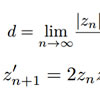﻿ Mathematics# Mathematics

### 2014

Force exerted by a band clamp How to calculate the total inward force exerted by hose clamps, band clamps etc.

### 2012

Distance estimation Distance estimation techniques - point to line, point to isoline, Mandelbrot/Julia sets

### 2004

Prismatic rings Some maths on prismatic rings and the relation to Celtic knotwork patterns